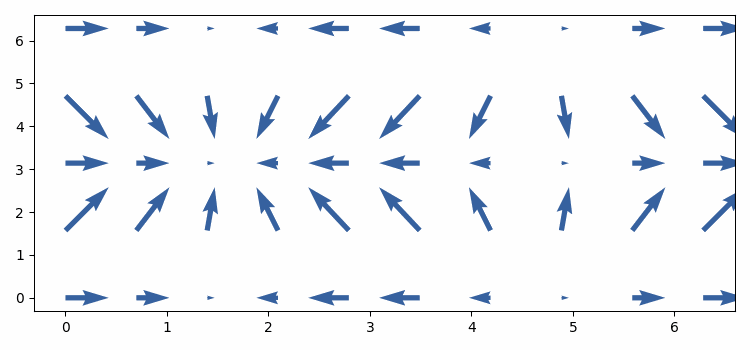# Plotting animated quivers in Python using Matplotlib

To animate quivers in Python, we can take the following steps −

• Set the figure size and adjust the padding between and around the subplots.
• Create x and y data points using numpy.
• Create u and v data points using numpy.
• Create a figure and a set of subplots.
• Plot a 2D field of arrows using quiver() method.
• To animate the quiver, we can change the u and v values, in animate() method. Update the u and v values and the color of the vectors.
• To display the figure, use show() method.

## Example

import numpy as np
import random as rd
from matplotlib import pyplot as plt, animation

plt.rcParams["figure.figsize"] = [7.50, 3.50]
plt.rcParams["figure.autolayout"] = True

x, y = np.mgrid[:2 * np.pi:10j, :2 * np.pi:5j]
u = np.cos(x)
v = np.sin(y)

fig, ax = plt.subplots(1, 1)
qr = ax.quiver(x, y, u, v, color='red')

def animate(num, qr, x, y):
u = np.cos(x + num * 0.1)
v = np.sin(y + num * 0.1)
qr.set_UVC(u, v)
qr.set_color((rd.random(), rd.random(), rd.random(), rd.random()))
return qr,

anim = animation.FuncAnimation(fig, animate, fargs=(qr, x, y),
interval=50, blit=False)

plt.show()

## Output